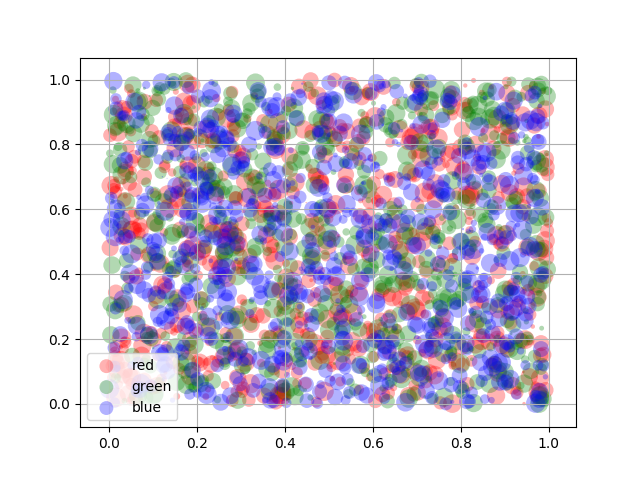Travis-CI:# Scatter plots with a legend¶

Also demonstrates how transparency of the markers can be adjusted by giving `alpha` a value between 0 and 1.```import matplotlib.pyplot as plt
from numpy.random import rand

fig, ax = plt.subplots()
for color in ['red', 'green', 'blue']:
n = 750
x, y = rand(2, n)
scale = 200.0 * rand(n)
ax.scatter(x, y, c=color, s=scale, label=color,
alpha=0.3, edgecolors='none')

ax.legend()
ax.grid(True)

plt.show()
```

Total running time of the script: ( 0 minutes 0.025 seconds)

Gallery generated by Sphinx-Gallery